Next: Mathematical Algorithms and Up: Computational Geometry and Previous: Computational Metrology of

Unfolding Measured Distributions

Bert W. Rust and Dianne P. O'Leary, ACMD

Many measured distributions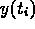are modeled by a system of integral equations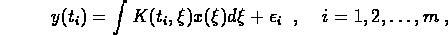where the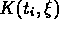are known, the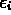are measuring errors, and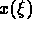is an unknown function to be determined. Since these integral equations are ubiquitous in measurement processes, methods for solving them are of great interest to NIST and ACMD.

Replacingby a discrete approximation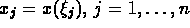, gives the linear regression model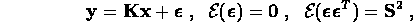where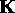is an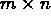matrix and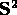is the covariance matrix for the measurements. The corresponding least squares problem is usually so poorly conditioned that the usual methods give wildly oscillating, unphysical solutions.

This project previously produced three algorithms, CLASIC, FERDIT and BRAKET-LS, which give estimates and confidence intervals for the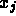. CLASIC uses a singular value decomposition (SVD) of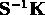to implement iterative refinement on the augmented system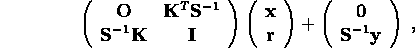with an option for truncating the SVD to suppress the amplification of measurement noise.

The FERDIT algorithm assumes that the solutionis nonnegative and iterates on a regularized regression model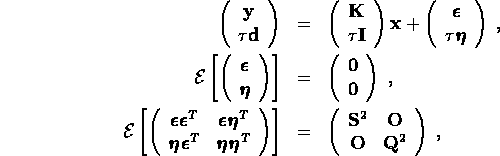where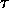is the regularization parameter,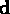is a positive vector and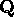is a related nonnegative diagonal matrix designed to constrain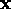to the positive orthant. The iteration is implemented with an SVD of the matrix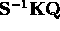. The bounds obtained are conservative and suboptimal, but are useful as starting estimates for the BRAKET-LS method.

The BRAKET-LS algorithm also assumes a nonnegative solution and uses a parametric quadratic programming procedure to produce rigorous confidence interval bounds defined by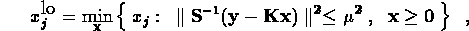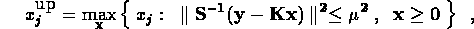where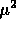is a constant chosen to give the desired confidence level. The method requires initial estimates which do not have to bracket thevery closely, but the computational effort is considerably reduced by using the suboptimal bounds obtained from the FERDIT method.

Current efforts have been devoted to simplifying and documenting the Fortran subroutines and writing a paper describing the BRAKET-LS software.Next: Mathematical Algorithms and Up: Computational Geometry and Previous: Computational Metrology of